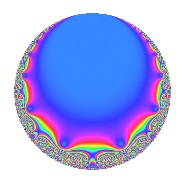# Properties

 Label 12.5.cLevel 12 Weight 5 Character orbit c Rep. character $$\chi_{12}(5,\cdot)$$ Character field $$\Q$$ Dimension 1 Newform subspaces 1 Sturm bound 10 Trace bound 0

# Related objects

## Defining parameters

 Level: $$N$$ = $$12 = 2^{2} \cdot 3$$ Weight: $$k$$ = $$5$$ Character orbit: $$[\chi]$$ = 12.c (of order $$2$$ and degree $$1$$) Character conductor: $$\operatorname{cond}(\chi)$$ = $$3$$ Character field: $$\Q$$ Newform subspaces: $$1$$ Sturm bound: $$10$$ Trace bound: $$0$$

## Dimensions

The following table gives the dimensions of various subspaces of $$M_{5}(12, [\chi])$$.

Total New Old
Modular forms 11 1 10
Cusp forms 5 1 4
Eisenstein series 6 0 6

## Trace form

 $$q + 9q^{3} - 94q^{7} + 81q^{9} + O(q^{10})$$ $$q + 9q^{3} - 94q^{7} + 81q^{9} + 146q^{13} - 46q^{19} - 846q^{21} + 625q^{25} + 729q^{27} + 194q^{31} - 2062q^{37} + 1314q^{39} - 3214q^{43} + 6435q^{49} - 414q^{57} - 1966q^{61} - 7614q^{63} + 5906q^{67} - 8542q^{73} + 5625q^{75} + 7682q^{79} + 6561q^{81} - 13724q^{91} + 1746q^{93} - 18814q^{97} + O(q^{100})$$

## Decomposition of $$S_{5}^{\mathrm{new}}(12, [\chi])$$ into newform subspaces

Label Dim. $$A$$ Field CM Traces $q$-expansion
$$a_2$$ $$a_3$$ $$a_5$$ $$a_7$$
12.5.c.a $$1$$ $$1.240$$ $$\Q$$ $$\Q(\sqrt{-3})$$ $$0$$ $$9$$ $$0$$ $$-94$$ $$q+9q^{3}-94q^{7}+3^{4}q^{9}+146q^{13}+\cdots$$

## Decomposition of $$S_{5}^{\mathrm{old}}(12, [\chi])$$ into lower level spaces

$$S_{5}^{\mathrm{old}}(12, [\chi]) \cong$$ $$S_{5}^{\mathrm{new}}(6, [\chi])$$$$^{\oplus 2}$$